## Inlining S-Functions

### Inlining an S-function

To inline an S-function means to provide a TLC file for an S-Function block that will replace the C, C++, Fortran, or MATLAB® language version of the block that was used during simulation.

### Noninlined S-Function

If an inlining TLC file is not provided, most targets support the block by recompiling the C MEX S-function for the block. As discussed earlier, there is overhead in memory usage and speed when using a C/C++ coded S-function and a limited subset of `mx*` API calls supported within the code generator context. If you want the most efficient generated code, you must inline S-functions by writing a TLC file for them.

When the simulation needs to execute one of the functions for an S-function block, it calls the MEX-file for that function. When the code generator executes a noninlined S-function, it does so in a similar manner, as this diagram illustrates.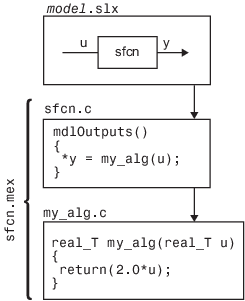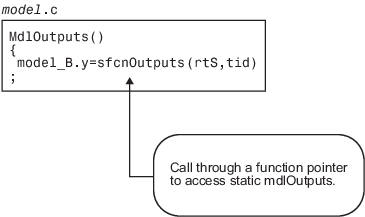### Types of Inlining

It is helpful to define two categories of inlining:

• Fully inlined S-functions

• Wrapper inlined S-functions

While both inline the S-function and remove the overhead of a noninlined S-function, the two approaches are different. The first example below, using `timestwo.tlc`, is considered a fully inlined TLC file, where the full implementation of the block is contained in the TLC file for the block.

The second example uses a wrapper TLC file. Instead of generating the algorithmic code in place, this example calls a C function that contains the body of code. There are several potential benefits for using the wrapper TLC file:

• It provides a way for the C MEX S-function and the generated code to share the C code. You do not need to write the code twice.

• The called C function is an optimized routine.

• Several of the blocks might exist in the model, and it is more efficient in terms of code size to have them call a function, as opposed to each creating identical algorithmic code.

• It provides a way to incorporate legacy C code seamlessly into generated code.

### Fully Inlined S-Function Example

Inlining an S-function provides a mechanism to directly embed code for an S-function block into the generated code for a model. Instead of calling into a separate source file via function pointers and maintaining a separate data structure (`SimStruct`) for it, the code appears “inlined” as the next figure shows.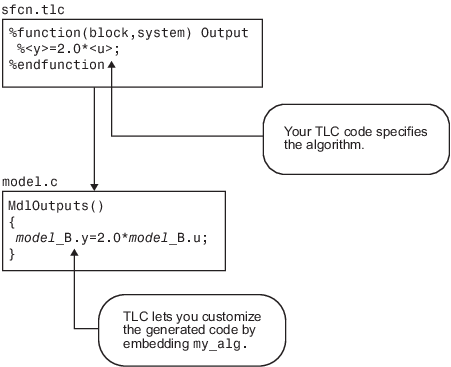The S-function `timestwo.c` provides a simple example of a fully inlined S-function. This block multiplies its input by 2 and outputs it. The C MEX version of the block is in the file `matlabroot/toolbox/simulink/simdemos/simfeatures/src/timestwo.c`, and the inlining TLC file for the block is `matlabroot/toolbox/simulink/simdemos/simfeatures/tlc_c/timestwo.tlc`.

#### timestwo.tlc

```%implements "timestwo" "C" %% Function: Outputs ========================================== %% %function Outputs(block, system) Output /* %<Type> Block: %<Name> */ %% /* Multiply input by two */ %assign rollVars = ["U", "Y"] %roll idx = RollRegions, lcv = RollThreshold, block, "Roller", rollVars %<LibBlockOutputSignal(0, "", lcv, idx)> = \ %<LibBlockInputSignal(0, "", lcv, idx)> * 2.0; %endroll %endfunction```

#### TLC Block Analysis

The `%implements` directive is required by TLC block files and is used by the Target Language Compiler to verify the block type and language supported by the block. The `%function` directive starts a function declaration and shows the name of the function, `Outputs`, and the arguments passed to it, `block` and `system`. These are the relevant records from the `model.rtw` file for this instance of the block.

The last piece of the prototype is `Output`. This means that any line that is not a TLC directive is output by the function to the current file that is selected in TLC. So, nondirective lines in the `Outputs` function become generated code for the block.

The most complicated piece of this TLC block example is the `%roll` directive. TLC uses this directive to provide automatic generation of `for` loops, depending on input/output widths and whether the inputs are contiguous in memory. This example uses the typical form of accessing outputs and inputs from within the body of the roll, using `LibBlockOutputSignal` and `LibBlockInputSignal` to access the outputs and inputs and perform the multiplication and assignment. Note that this TLC file supports any valid signal width.

The only function used to implement this block is `Outputs`. For more complicated blocks, other functions are declared as well. You can find examples of more complicated inlining TLC files in the folders `matlabroot/toolbox/simulink/simdemos/simfeatures/tlc_c` (open) and `matlabroot/toolbox/simulink/blocks/tlc_c` (open), and by looking at the code for built-in blocks in the folder `matlabroot/rtw/c/tlc/blocks` (open).

#### The timestwo Model

This simple model uses the `timestwo` S-function and shows the `MdlOutputs` function from the generated `model.c` file, which contains the inlined S-function code.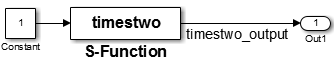#### Model Outputs Code

```/* Model output function */ static void timestwo_ex_output(int_T tid) { /* S-Function Block: <Root>/S-Function */ /* Multiply input by two */ timestwo_ex_B.timestwo_output = timestwo_ex_P.Constant_Value * 2.0; /* Outport: '<Root>/Out1' */ timestwo_ex_Y.Out1 = timestwo_ex_B.timestwo_output; }```

### Wrapper Inlined S-Function Example

The following diagram illustrates inlining an S-function as a wrapper. The algorithm is directly called from the generated model code, removing the S-function overhead but maintaining the user function.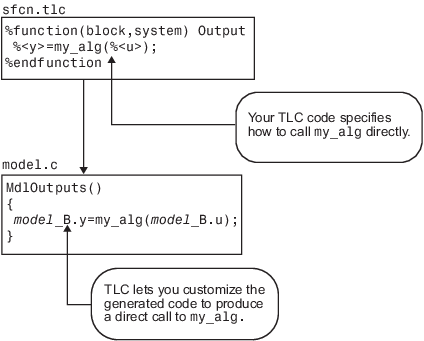This is the inlining TLC file for a wrapper version of the `timestwo` block.

```%implements "timestwo" "C" %% Function: BlockTypeSetup ================================== %% %function BlockTypeSetup(block, system) void %% Add function prototype to model's header file %<LibCacheFunctionPrototype... ("extern void mytimestwo(real_T* in,real_T* out,int_T els);")> %% Add file that contains "myfile" to list of files to be compiled %<LibAddToModelSources("myfile")> %endfunction %% Function: Outputs ========================================== %% %function Outputs(block, system) Output /* %<Type> Block: %<Name> */ %assign outPtr = LibBlockOutputSignalAddr(0, "", "", 0) %assign inPtr = LibBlockInputSignalAddr(0, "", "",0) %assign numEls = LibBlockOutputSignalWidth(0) /* Multiply input by two */ mytimestwo(%<inPtr>,%<outPtr>,%<numEls>); %endfunction```

#### Analysis

The function `BlockTypeSetup` is called once for each type of block in a model; it doesn't produce output directly like the `Outputs` function. Use `BlockTypeSetup` to include a function prototype in the `model.h` file and to tell the build process to compile an additional file, `myfile.c`.

Instead of performing the multiplication directly, the `Outputs` function now calls the function `mytimestwo`. All instances of this block in the model call the same function to perform the multiplication. The resulting model function, `MdlOutputs`, then becomes

```static void timestwo_ex_output(int_T tid) { /* S-Function Block: <Root>/S-Function */ /* Multiply input by two */ mytimestwo(&model_B.Constant_Value,&model_B.S_Function,1); /* Outport Block: <Root>/Out1 */ model_Y.Out1 = model_B.S_Function; }```